﻿ Mechanics Map - Engineering Structures

# Engineering Structures

An engineering structure is a term used to describe any set of interconnected bodies. The different bodies in the structure can move relative to one another (such as the blades in a pair of scissors) or they can be be fixed relative to one another (such as the different beams connected to form a bridge).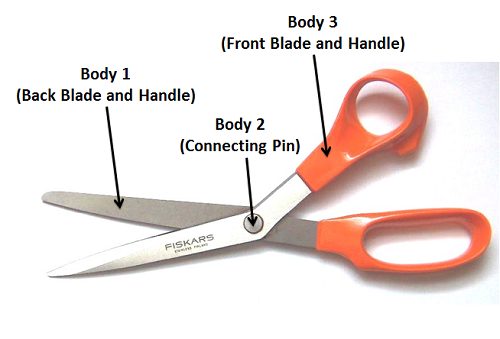This pair of scissors is an example of an engineering structure. The structure consists of three different bodies that are interconnected. Adapted from Public Domain image by Comet27.

When analyzing engineering structures, sometimes we will analyze the structure as a whole, and sometimes we will break the structure down into individual components that are analyzed separately. The exact methods used depend upon what unknown forces we are looking for and what type of structure we are analyzing.

## Internal and External Forces:

When examining a single body, we would find the forces that this body exerted on the bodies around it and the forces that these surrounding bodies would exert back on the body we are analyzing. These forces are all considered external forces because they are forces acting between the body and the external environment.

In an engineering structure, we still have external forces where the structure is interacting with bodies and enviroment external to the structure, but we also can think about the forces that different components in the structure exert on one another (the forces between the pin and the blade in the diagram above for example). Since both of these bodies are part of the structure we are analyzing, this forces are considered internal forces.

If we only wish to determine the external forces acting on a structure, then we can treat the whole structure as a single body (assuming the structure is rigid as a whole). If we wish to determine the internal forces acting between components in the structure, then we will need to disassemble the structure into separate bodies in our analysis.

## Types of Structures:

Another important consideration when analyzing structures is the type of structure that is being analyzed. All structures fall into one of three categories: trusses, frames, or machines. Frames and machines are analyzed in the same way so distinction between them is less important, but the analysis methods used for trusses vary greatly from the analysis methods used for frames and machines, so determining if a structure is a truss or not is an important first step in structure analysis.

### Trusses:

A truss is a structure that consists entirely of two force members. If any one body in the structure is not a two force member then the structure is either a frame or a machine. Also in order to be a statically determinate truss (a truss where we can actually solve for all the unknowns) the truss must be independently rigid as a whole. If different parts of the truss could move relative to one another then the truss separated is not independently rigid.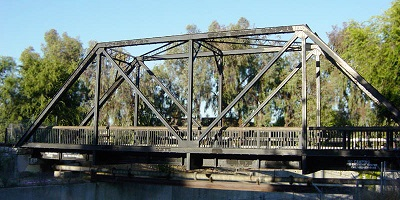This bridge is an example of a truss. It consists of a number of members connected at only two points (the ends of the beams). Public domain image by Leonard G.

A two force member is a body where forces are applied at only two locations. If forces are applied at more than two locations, or if any moments are applied then the body is not a two force member (see the two force member page for more details). Because of the unique assumptions we can make with two force members, we can apply two unique methods to the analysis of trusses (the method of joints and the method of sections) that we cannot apply to frames and machines (where we cannot assume we have two force members).

Trusses can be broken down further into plane trusses and space trusses. A plane truss is a truss where all members lie in a single plane. This means that plane trusses can essentially be treated as two dimensional systems. Space trusses on the other hand have members that are not limited to a single plane. This means that space trusses need to be analyzed as a three dimensional system.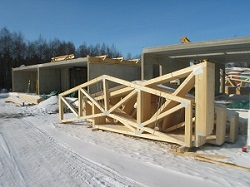The members of these trusses all lie in a single plane. These roof trusses are an example of a plane truss. Image by Riisipuuro CC-BY-SA 3.0.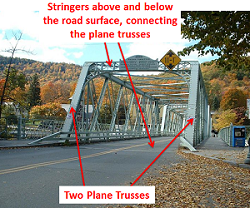This bridge consists of two plane trusses connected by members called stringers. Adapted from image by ToddC4176 CC-BY-SA 3.0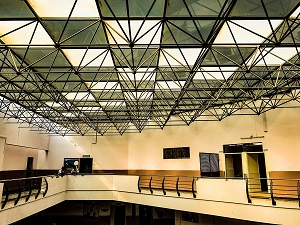This roof supporting truss does not lie in a single plane. This is an example of a space truss. Image by IM3847 CC-BY-SA 4.0.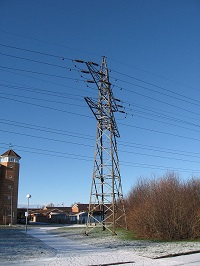The power line tower also does not lie in a single plane and is therefore a space truss. Image by Anders Lagerås CC-BY-SA 2.5.

### Frames and Machines:

A frame or a machine is structure where at least one component of the structure is not a two force member. This will be a component in the structure that has forces acting at three or more points on the body. This could be three connection points on the body, or it could be two connection points, with a force applied at a third location.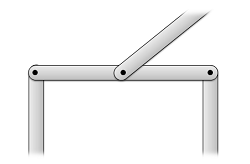This beam is connected to other members (where normal forces would exist) at more than two locations. This beam is therefore not a two force member.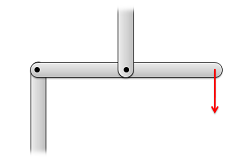This beam has two connection points, but a force is acting on a third point. Therefore the beam has forces acting on it at more than two locations and it is not a two force member.

In terms of the difference between a frame and a machine, a frame is a rigid structure as a whole, while a machine is not rigid. This means that no part can move relative to the other parts in a frame, while parts can move relative to one another in a machine. Though there is a difference in vocabulary in describing frames and machines, they are grouped together here because we use the same process to analyze both of these structures.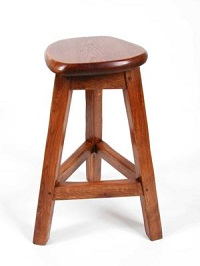The legs of this stool have forces applied to them in three locations (the top, the cross beams, and the floor). The stool is also independently rigid so this is a frame. Image by Besceh31 CC-BY-SA 2.5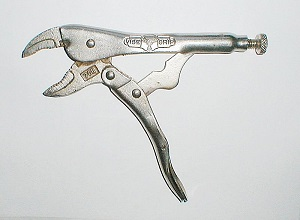Many pieces within this pair of locking pliers have forces applied to them at more than two locations. The pieces can also move relative to one another so this is an example of a machine. Image by Duk CC-BY-SA 3.0

Because frames and machines do not consist entirely of two force members, we cannot make the assumptions that allow us to use the method of joints and the method of sections. For this reason, we need to use a different analysis method (simply called the analysis of frames and machines here).